# Bonbons

Create a mixture of 50 kg of candy on price 700Kč. Candies has prices: 820Kč, 660Kč and 580Kč. Use cross rule.

Correct result:

a =  15 kg
b =  30 kg
c =  5 kg

#### Solution:

a+b+c=50
a=15
820a+660b+580c = 50•700

a+b+c=50
a=15
820•a+660•b+580•c = 50•700

a+b+c = 50
a = 15
820a+660b+580c = 35000

a = 15
b = 30
c = 5

Our linear equations calculator calculates it.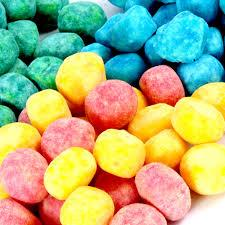We would be pleased if you find an error in the word problem, spelling mistakes, or inaccuracies and send it to us. Thank you!Tips to related online calculators
Do you have a system of equations and looking for calculator system of linear equations?
Most natural application of trigonometry and trigonometric functions is a calculation of the triangles. Common and less common calculations of different types of triangles offers our triangle calculator. Word trigonometry comes from Greek and literally means triangle calculation.

## Next similar math problems:

• CoffeeIn stock are three kinds of branded coffee prices: I. kind......205 Kc/kg II. kind......274 Kc/kg III. kind.....168 Kc/kg Mixing these three species in the ratio 8:5:6 create a mixture. What will be the price of 100 grams of this mixture?
• CandiesThe price for 1 kg of more expensive candies is 125 CZK. The price for 1 kg of cheaper candy is 100 CZK. We mix two different mixtures of candy. A) The first mixture contains 2 kg more expensive and 0.5 kg cheaper candy. Calculate the price for 1 kg of th
• Alcohol mixtureFrom 55% and 80% alcohol we have to produce 0.2 kg of 60% alcohol. How many of them do we use in solution?
• MixingIf we mix 5 kg of goods of one kind and 3 kg second one, resulting mixture cost 16.50 EUR/kg. If these quantities are mixed in reverse - first three kilograms and 5 kilograms second cost of mixture is 18.50 EUR/kg. What is the price of one kg of goods of
• Bonbons 2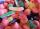Kilo sweets will cost 260 CZK. The first type has a price per 320 kg, the second type 240 CZK per kg. How many kilos of both kinds of sweets need to prepare a 100 kg mixture ?
• SweetsWe want to prepare 5 kg of sweets for 150 CZK. We will mix cheaper candy: 1 kg for 120 CZK and more expensive candy: 1 kg per 240 CZK. How much of this two types of candy is necessary to prepare this mixture?
• Cork and swimmingIf a person weighs 80 kg, how many kilograms of cork must take swimming belt to use it to float on water? The density of the human body is 1050kg/m3 and cork 300kg/m3. (Instructions: Let the human body and cork on a mixture that has a density of 1000kg/m3
• Two teas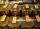A mixture weighing 10 kg was made from two types of tea. The price of tea was 160 CZK/kg and second tea 170 CZK/kg. The price of the mixture is 166 CZK/kg. How many kilograms of each type of tea had to be mixed?
• Coffee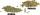Coffee merchant has coffee robusta and arabica species. 1 kg Robusta worth 450 CZK, Arabica 1 kg is 300 CZK more expensive. Calculate how many kilograms of Robusta and Arabica will need to produce 30 kg of the mixture so that the mixture cost is 490 CZK p
• Mixture of nuts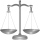Alice bought a mixture of nuts containing cashew nuts, hazelnuts and peanuts in a ratio of 1: 2: 3. Calculate the weight in grams of the whole mixture if the peanuts weigh 90 g.
• SpiritFrom 55% and 80% spirit we would like to produce 0.2 kg of 60% spirit. How many of them we must use in a solution?
• ApplesSchool kitchen bought 36kg apples for 12 kc/kg. How many kilograms of apples 1/4 cheaper can they buy for the same money?
• CandyThe price for 1kg of more expensive candy is 125 CZK. The price for 1kg of cheaper candy is 100 CZK. We make two different blends of candy. And now. The second blend contains 2kg of more expensive candy and several kg of cheaper candy. The price per 1 kg
• Tea mixture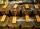Of the two sort of tea at a price of 180 CZK/kg and 240 CZK/kg we make a mixture 12 kg that should be prepared at a price of 200 CZK / kg. How many kilos of each sort of tea will we need to be mixed?
• Candies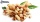In the confectionery, the price for 1 kg of pistachio candies cost CZK 360, and the price for 1 kg of hazelnut candies was CZK 280. Mixing these two types of sweets created a box of chocolates. How many grams of pistachios and how many grams of hazelnut c
• Tea blendTea blends are maked from two kinds of tea. In standard tea mixture are two teas in the ratio 1:3 and 40 g costs 42 CZK. In the premium tea mixture are weighing two teas in the ratio 1:1 and 50 grams costs 60 CZK. How much cost 10 grams of more expensive
• Metal alloy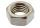What is the ratio of metals in the alloy that is in the 50 tonnes of steel to 30 kg nickel?